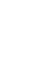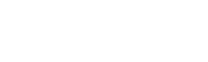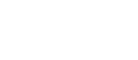What Are Rational Numbers?

What are rational numbers? Rational numbers are numbers that can be expressed as a fraction. In this fraction, both the numerator and denominator must be integers and the denominator cannot be 0. Let’s look at some examples of rational numbers to help you better classify them.

Natural Numbers

Natural numbers are numbers used for counting and ordering. They are positive integers, beginning with 1. Therefore, some examples include: 1, 2, 3, 4, 5 . . .

Whole Numbers

Whole numbers include all natural numbers with one big difference: it also includes 0. So, when you’re listing whole numbers, you could say 0, 1, 2, 3, 4 . . .

Integers

Integers include all natural and whole numbers. The thing that sets integers apart from these first two groups is that they also include negative numbers. Since integers are rational numbers, you can be assured that the negative counterparts of natural and whole numbers are considered rational, too. Examples of integers include . . . -5, -4, -3, -2, -1, 0, 1, 2, 3, 4, 5 . . .

Proper Fractions

Proper fractions are numbers that are less than 1. Examples of fractions includeYou can think of these numbers as parts of a whole, such as a slice of pie. And, naturally, these numbers are expressed as fractions, so they easily fit the bill as rational numbers.

Improper Fractions

Proper fractions are numbers less than 1, and improper fractions are numbers that are greater than 1. Maybe you have an entire pie plus an addition half of a pie available. In this case, you could write the fraction as:Other examples of improper fractions include:Mixed Fractions

You can convert improper fractions into mixed fractions. Mixed fractions include both proper fractions and whole numbers. If we look at the examples from the improper fractions, we can turn these into mixed numbers:Decimal Numbers

Decimal numbers also express values less than 1. Maybe the baby weighed 6.5 pounds at birth. The decimal tells us that the baby weighed an extra half of a pound. In between the numbers is a decimal point, which is similar to a period. This tells us that all the numbers after the decimal point are a percentage of the whole. And, of course, this is an integer that could be written as a fraction:which means this would still be considered a rational number.

Some people wonder about repeating decimal numbers. Are these rational numbers? The answer is yes. One of the most common ones that people face is .6666666 with the 6 repeating infinitely. Although it doesn’t seem like it’s a real number, it is. Why? Because it can be expressed as a fraction: 2/3.

Irrational Numbers

It seems like every number is covered as a rational number, right? So, what is an irrational number? An irrational number can’t be expressed as a fraction and is not an imaginary number. One of the most famous irrational numbers is pi. This number is typically shown as 3.14159…., but the number continues on without repeating. Other numbers that are irrational include:When you look at a set of numbers, try to figure out which are rational numbers and which are irrational. As you look to classify a number as either rational or irrational, be sure to consider whether or not you can express the number as a fraction. If it’s an irrational number, you’re going to have an infinite amount of digits to work with, which may make you feel a little irrational, too!

Author

•Jamie graduated from Brigham Young University- Idaho with a degree in English Education. She spent several years teaching and tutoring students at the elementary, high school, and college level. She currently works as a contract writer and curriculum developer for online education courses. In her free time, she enjoys running and spending time with her boys!